# SSC (Marathi Semi-English) 10th Standard [इयत्ता १० वी] - Maharashtra State Board Question Bank Solutions for Geometry

Subjects
Topics
Subjects
Popular subjects
Topics
Geometry
< prev  1 to 20 of 1220  next >

In the following figure, in ΔPQR, seg RS is the bisector of ∠PRQ. If PS = 6, SQ = 8, PR = 15, find QR.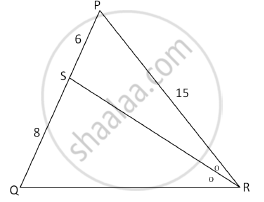[0.01] Similarity
Chapter: [0.01] Similarity
Concept: Similarity of Triangles

ΔSHR ~ ΔSVU. In ΔSHR, SH = 4.5 cm, HR = 5.2 cm, SR = 5.8 cm and "SH"/("SV")=3/5. Construct ΔSVU.

[0.04] Geometric Constructions
Chapter: [0.04] Geometric Constructions
Concept: Basic Geometric Constructions

Draw ∠ABC of measure 105° and bisect it.

[0.04] Geometric Constructions
Chapter: [0.04] Geometric Constructions
Concept: Basic Geometric Constructions

In the following figure, in Δ PQR, seg RS is the bisector of ∠PRQ.

PS = 3, SQ = 9, PR = 18. Find QR.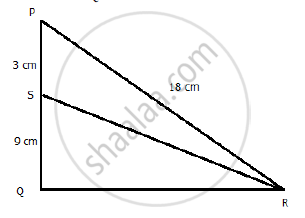[0.01] Similarity
Chapter: [0.01] Similarity
Concept: Similarity of Triangles

Prove that the angle bisector of a triangle divides the side opposite to the angle in the ratio of the remaining sides.

[0.01] Similarity
Chapter: [0.01] Similarity
Concept: Similarity of Triangles

In the figure given below, Ray PT is bisector of ∠QPR. If PQ = 5.6 cm, QT = 4 cm and TR = 5 cm, find the value of x .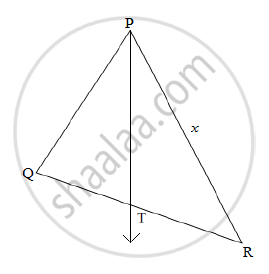[0.01] Similarity
Chapter: [0.01] Similarity
Concept: Similarity of Triangles

In a triangle ABC, line l || Side BC and line l intersects side AB and AC in points P and Q, respectively. Prove that: "AP"/"BP"="AQ"/"QC"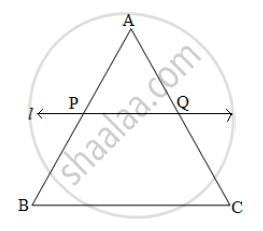[0.01] Similarity
Chapter: [0.01] Similarity
Concept: Similarity of Triangles

Prove that the points (3, 0), (6, 4) and (-1, 3) are the vertices of a right-angled isosceles triangle.

[0.05] Co-ordinate Geometry
Chapter: [0.05] Co-ordinate Geometry
Concept: Coordinate Geometry

In the given figure, two chords AB and CD of a circle intersect each other at the point P (when produced) outside the circle. Prove that

(i) ΔPAC ∼ ΔPDB

(ii) PA.PB = PC.PD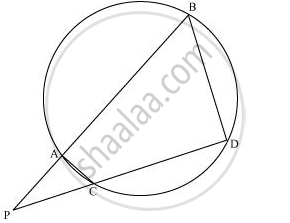[0.01] Similarity
Chapter: [0.01] Similarity
Concept: Similarity of Triangles

triangleDEF ~ triangleMNK. If DE = 5 and MN = 6, then find the value of (A(triangleDEF))/(A(triangleMNK))

[0.01] Similarity
Chapter: [0.01] Similarity
Concept: Similarity of Triangles

Draw angle ABC of measure 80° and bisect it

[0.04] Geometric Constructions
Chapter: [0.04] Geometric Constructions
Concept: Basic Geometric Constructions

In the following figure, seg DH ⊥ seg EF and seg GK ⊥ seg EF. If DH = 18 cm, GK = 30 cm and A(triangle DEF) = 450 cm^2, then find:

1) EF

2) A(triangle GFE)

3) A(square DFGE)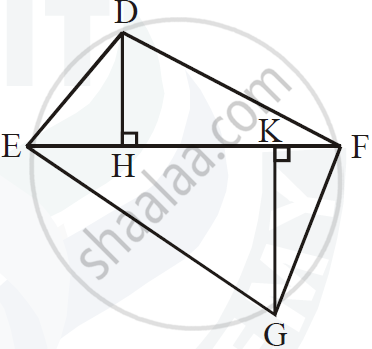[0.01] Similarity
Chapter: [0.01] Similarity
Concept: Similarity of Triangles

On which axis do the following points lie?

R(−4,0)

[0.05] Co-ordinate Geometry
Chapter: [0.05] Co-ordinate Geometry
Concept: Coordinate Geometry

In what ratio is the line segment joining (-3, -1) and (-8, -9) divided at the point (-5, -21/5)?

[0.05] Co-ordinate Geometry
Chapter: [0.05] Co-ordinate Geometry
Concept: Coordinate Geometry

In the following figure, seg BE ⊥ seg AB and seg BA ⊥ seg AD. If BE = 6 and $\text{AD} = 9 \text{ find} \frac{A\left( \Delta ABE \right)}{A\left( \Delta BAD \right)} \cdot$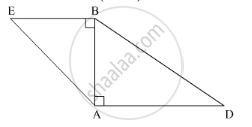[0.01] Similarity
Chapter: [0.01] Similarity
Concept: Similarity of Triangles

Draw ∠ABC of measures 135°and bisect it.

[0.04] Geometric Constructions
Chapter: [0.04] Geometric Constructions
Concept: Basic Geometric Constructions

In the given figure, X is any point in the interior of triangle. Point X is joined to vertices of triangle. Seg PQ || seg DE, seg QR || seg EF. Fill in the blanks to prove that, seg PR || seg DF.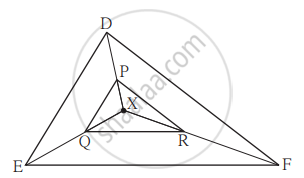Proof :  In ΔXDE, PQ || DE         ...square

∴ "XP"/square = square/"QE"                               ...(I) (Basic proportionality theorem)

In ΔXEF, QR || EF                       ...square

∴ square/square = square/square                                                           ..."(II)" square

∴ square/square = square/square                                ...from (I) and (II)

∴ seg PR || seg DE           ...(converse of basic proportionality theorem)

[0.01] Similarity
Chapter: [0.01] Similarity
Concept: Similarity of Triangles

In ∆ABC, ray BD bisects ∠ABC and ray CE bisects ∠ACB. If seg AB ≅ seg AC then prove that ED || BC.

[0.01] Similarity
Chapter: [0.01] Similarity
Concept: Similarity of Triangles

In the given figure, ∠ABC = 75°, ∠EDC = 75° state which two triangles are similar and by which test? Also write the similarity of these two triangles by a proper one to one correspondence.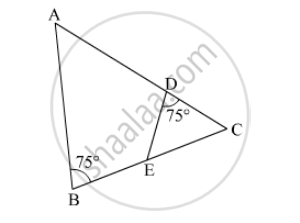[0.01] Similarity
Chapter: [0.01] Similarity
Concept: Similarity of Triangles

Are the triangles in the given figure similar? If yes, by which test?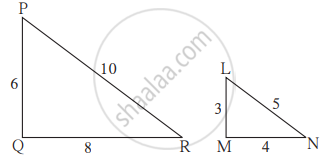[0.01] Similarity
Chapter: [0.01] Similarity
Concept: Similarity of Triangles
< prev  1 to 20 of 1220  next >